# 神经网络在交易中的实际应用。 是时候进行实践了

9 十月 2020, 06:571
4 265

###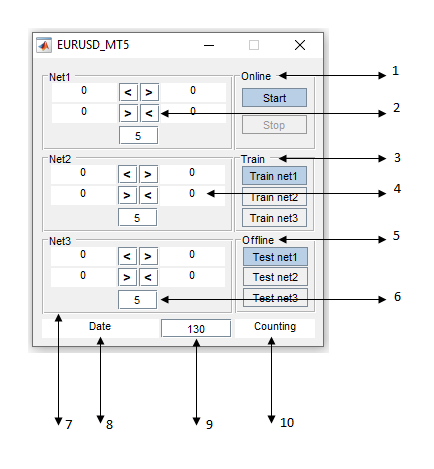1. Online 模块设计用于实际交易期间，以及在可视模式下进行测试时启动和停止神经网络。
2. 激活 Online 模块时，信息字段所含条件是信号曲线与神经网络响应曲线交叉。
3. "Train" 是一个演示模块，旨在训练神经网络和“再次训练”（？）。
4. 用于输出神经网络响应值的字段。 左侧 - 神经网络响应; 右侧 - 信号线。 下侧 - 当前柱线；上侧 - 前根柱线。
5. Offline 模块设计用于把神经网络针对测试样本的响应输出到数组。
6. 使用 Online 模块时，用于输入神经网络响应曲线平均值的字段。 (信号线周期)。 可编辑的值。
7. 模块 "Net1,2,3" — 在时间序列的不同时段中训练的三个网络子模块。 每个模块包括两个神经网络。
8. 使用 Online模块时，NNM 操作结束时间。
9. 使用 Online 模块时，以小时为单位输入 NNM 运行时间的字段。 可编辑的值。
10. 使用 Online 模块时，计算自 NNM 启动以来所经历的时间。

### 附件

1. MyAppInstaller_mcr.exe — 安装文件。
2. EURUSD_MT5.exe — 神经网络模块本身。
3. EURUSD_MT5var.exe — 神经网络模块的一个变体。
4. net1-net6.mat — 三个神经网络子网，即 Net1-Net3。 用作训练和测试的示例。
1. ExpertMatlabPodkach_MT5.mq5 和 ExpertMatlab_MT5.mq5 — 为 NNM 离线测试准备所需历史数据的两个 EA。
2. NWI.mq5 — 直观显示 NNM 响应的指标。
3. Matlab_MT5.mq5 — 在策略测试器中测试和优化 NNM 响应的 EA。
4. ExpertMatlabReal_MT5.mq5 — 实盘或模拟帐户在线操作以及在可视化模式进行测试的 EA。
5. EURUSDData.csv — 包含训练数据的文件。

### 程序安装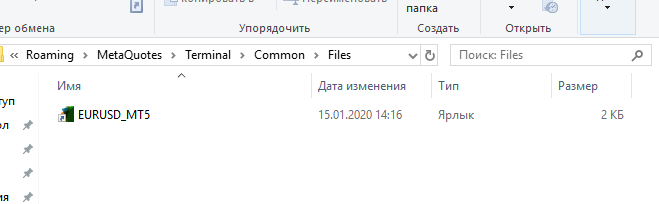1. 快捷 EURUSD_MT5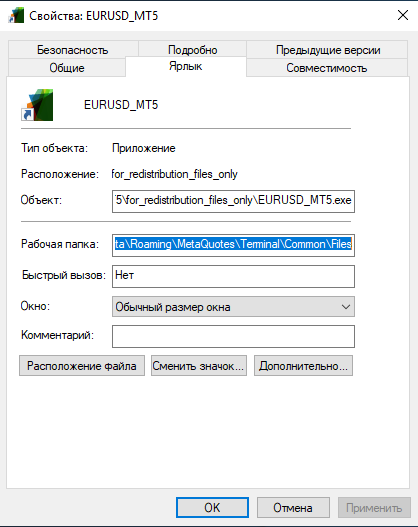2. 指定路径

### 实际运用

1. 在模拟或真实账户上进行在线交易
2. 在可视模式下测试 NNM
3. 神经网络训练
4. 从神经网络模块接收响应，并据其优化交易策略

1. 在模拟或真实账户里进行在线交易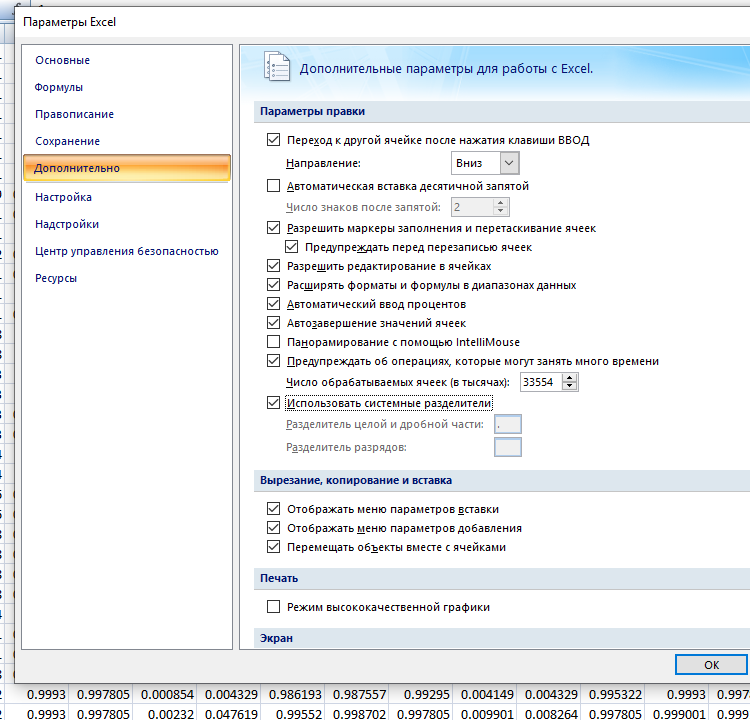3. Excel 参数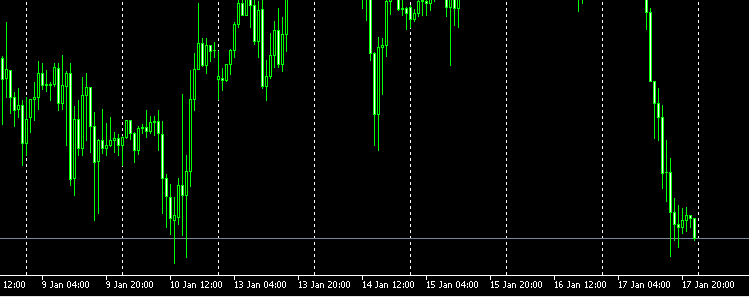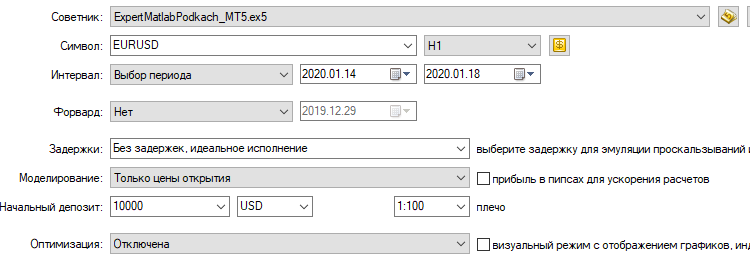4. 启动 ExpertMatlabPodkach_MT5.ex5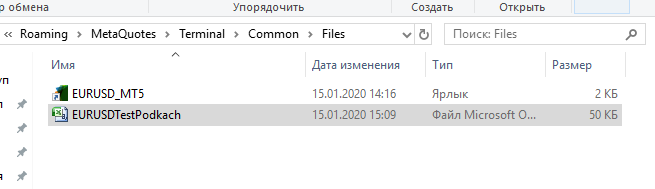5. 文件 EURUSDTestPodkach.csv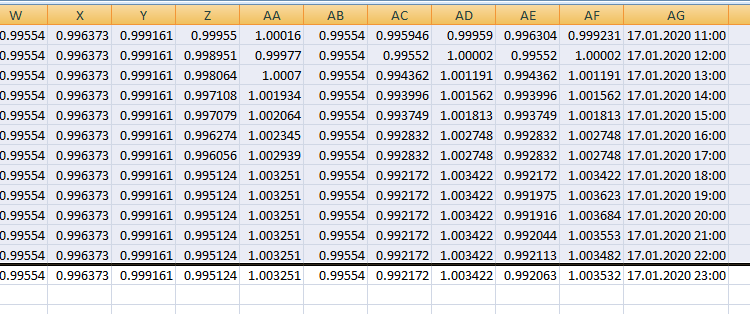6. 删除不必要的行

```#include<Trade\Trade.mqh>
//--- An object for performing trading operations

input int LossSell;
input int ProfitSell;

int BarMax;
int BarMin;
int handleInput;
int handleInputPodkach;
int handleBar;
int Con;
int Bar;

double DibMax;
double DibMin;

double in;

int Order01;
int Order1;

ulong TicketSell0;

bool send1;
bool send0;

//+------------------------------------------------------------------+
//| Expert initialization function                                   |
//+------------------------------------------------------------------+
int OnInit()
{
//---

if(handleInputPodkach==INVALID_HANDLE)

FileClose(handleInputPodkach);

//--- Setting MagicNumber to identify EA's orders
int MagicNumber=123456;
//--- Setting allowable slippage in points for buying/selling
int deviation=10;
//--- order filling mode, use the mode that is allowed by the server
//--- The function to be used for trading: true - OrderSendAsync(), false - OrderSend()

//---
return(INIT_SUCCEEDED);
}
//+------------------------------------------------------------------+
//| Expert deinitialization function                                 |
//+------------------------------------------------------------------+
void OnDeinit(const int reason)
{
//---
FileClose(handleInput);
FileClose(handleBar);
}

//+------------------------------------------------------------------+
//| Expert tick function                                             |
//+------------------------------------------------------------------+
void OnTick()
{
MqlDateTime stm;
TimeToStruct(TimeCurrent(),stm);

if(stm.hour==1)
DibMax=iHigh(NULL,PERIOD_H1,1);
if(stm.hour>0)
{
if(iHigh(NULL,PERIOD_H1,1)>DibMax && iTime(NULL,PERIOD_H1,0)>1)
{
in=iOpen(NULL,PERIOD_D1,0)-iLow(NULL,PERIOD_H1,1);
in=iHigh(NULL,PERIOD_H1,1)-iOpen(NULL,PERIOD_D1,0);
in=iHigh(NULL,PERIOD_D1,1)-iLow(NULL,PERIOD_D1,1);
in=iHigh(NULL,PERIOD_D1,1)-iOpen(NULL,PERIOD_H1,0);
in=iOpen(NULL,PERIOD_H1,0)-iLow(NULL,PERIOD_D1,1);
}
}
if(iHigh(NULL,PERIOD_H1,1)>DibMax)
DibMax=iHigh(NULL,PERIOD_H1,1);
//+------------------------------------------------------------------+
//|                                                                  |
//+------------------------------------------------------------------+
if(stm.hour==1)
DibMin=iLow(NULL,PERIOD_H1,1);
if(stm.hour>0)
{
if(iLow(NULL,PERIOD_H1,1)<DibMin && iTime(NULL,PERIOD_H1,0)>1)
{
in=iOpen(NULL,PERIOD_D1,0)-iLow(NULL,PERIOD_H1,1);
in=iHigh(NULL,PERIOD_H1,1)-iOpen(NULL,PERIOD_D1,0);
in=iHigh(NULL,PERIOD_D1,1)-iLow(NULL,PERIOD_D1,1);
in=iHigh(NULL,PERIOD_D1,1)-iOpen(NULL,PERIOD_H1,0);
in=iOpen(NULL,PERIOD_H1,0)-iLow(NULL,PERIOD_D1,1);
}
}
if(iLow(NULL,PERIOD_H1,1)<DibMin)
DibMin=iLow(NULL,PERIOD_H1,1);

in=iHigh(NULL,PERIOD_D1,1)-iOpen(NULL,PERIOD_H1,0);
in=iOpen(NULL,PERIOD_H1,0)-iLow(NULL,PERIOD_D1,1);

if(Bar<Bars(NULL,PERIOD_H1)&& stm.hour==0)
{
for(int i=19; i>=10; i--)
{
in[i-10]=in[i];
}

for(int i=29; i>=20; i--)
{
in[i-10]=in[i];
}
}

FileWrite(handleInput,

1/(in+1),1/(in+1),1/(in+1),1/(in+1),1/(in+1),1/(in+1),1/(in+1),1/(in+1),1/(in+1),1/(in+1),1/(in+1),1/(in+1),1/(in+1),1/(in+1),1/(in+1),1/(in+1),
1/(in+1),1/(in+1),1/(in+1),1/(in+1),1/(in+1),1/(in+1),1/(in+1),1/(in+1),1/(in+1),1/(in+1),1/(in+1),1/(in+1),1/(in+1),1/(in+1),1/(in+1),1/(in+1));

FileClose(handleInput);

FileWrite(handleBar,stm.hour);

FileClose(handleBar);

FileClose(Order01);

int    digits=(int)SymbolInfoInteger(_Symbol,SYMBOL_DIGITS);
double point=SymbolInfoDouble(_Symbol,SYMBOL_POINT);
double PriceBid=SymbolInfoDouble(_Symbol,SYMBOL_BID);

double TP0=NormalizeDouble(PriceBid-ProfitSell*point,digits);

if(Bar<Bars(NULL,PERIOD_H1))
Con=0;

Comment(Order1,"  ",Con);

SL1=0;

TP1=0;

if(LossSell==0)
SL0=0;

if(ProfitSell==0)
TP0=0;

if(Order1==0 && Bar<Bars(NULL,PERIOD_H1) && Con==0)
send0=true;

if(Order1==1 && Bar<Bars(NULL,PERIOD_H1) && Con==0)
send1=true;

if(send1==false  &&  Bar==Bars(NULL,PERIOD_H1) && Order1==1 && Con>=1 && iLow(NULL,PERIOD_H1,1)<iLow(NULL,PERIOD_H1,2) && stm.hour>15 && stm.hour<20)
{
}

if(send1==true &&  Bar==Bars(NULL,PERIOD_H1) && Order1==0 && Con>=1 && iHigh(NULL,PERIOD_H1,1)>iHigh(NULL,PERIOD_H1,2))
{
send1=false;
}
//---------Sell0
if(send0==false  &&  Bar==Bars(NULL,PERIOD_H1) && Order1==0 && Con>=1 && iHigh(NULL,PERIOD_H1,1)>iHigh(NULL,PERIOD_H1,2) && stm.hour>
11 && stm.hour<14)
{
}

if(send0==true &&  Bar==Bars(NULL,PERIOD_H1) && Order1==1 && Con>=1 && iLow(NULL,PERIOD_H1,1)<iLow(NULL,PERIOD_H1,2))
{
send0=false;
}
//-----------------------------------------------------------------------
Bar=Bars(NULL,PERIOD_H1);
Con++;
}
//------------------------------------------------------------------------

```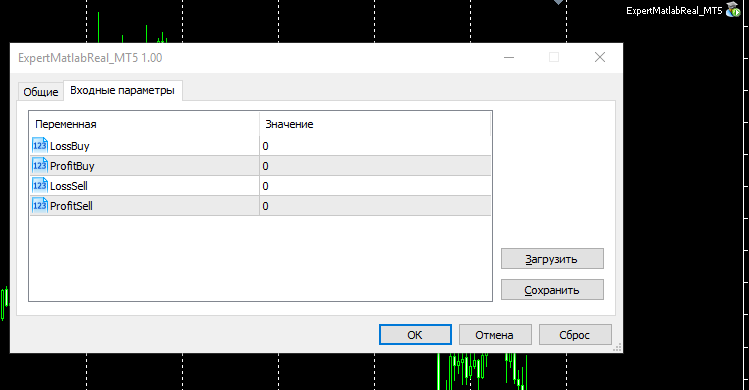7. 启动 ExpertMatlabReal_MT5.ex5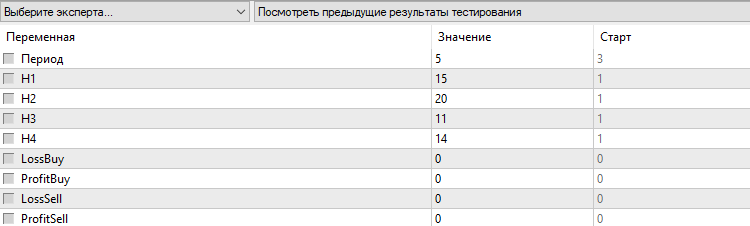7.1 Matlab_MT5.ex5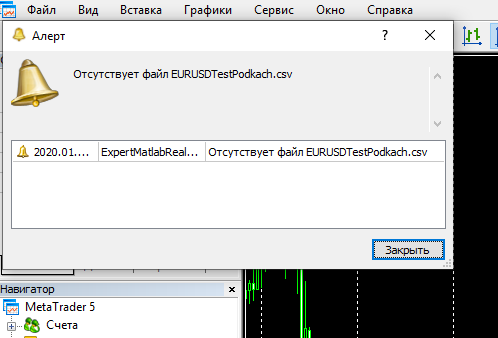8. 警告

EA 会将两个文件写入 ...\Common\Files 文件夹。 Input_mat.txt 文件包含 NNM 的输入数据。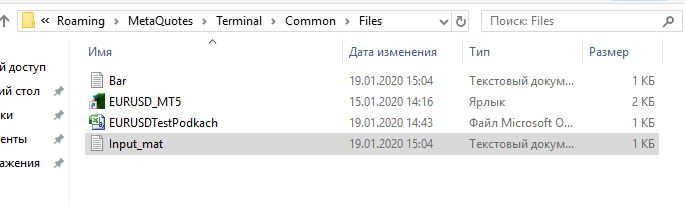9. 智能交易系统响应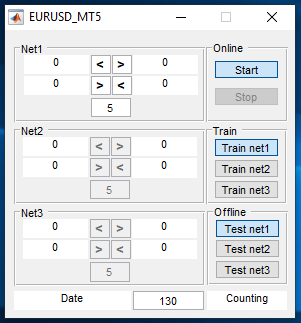10. NSM 工作空间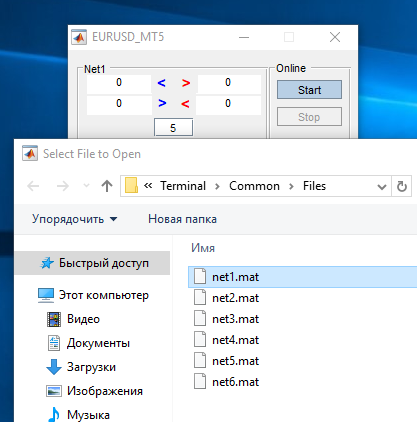11. 按下 "Start"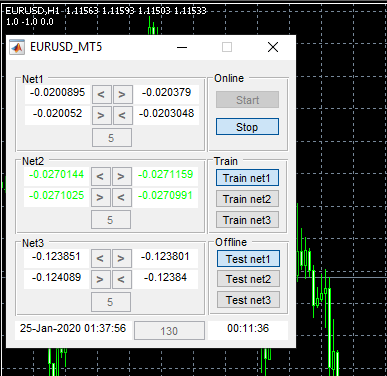12. NNM 处于交易状态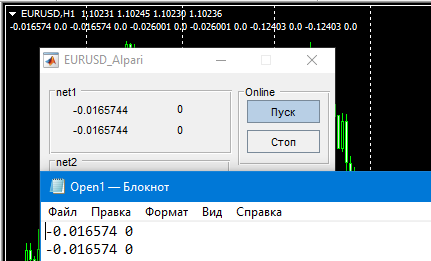13. 信息控制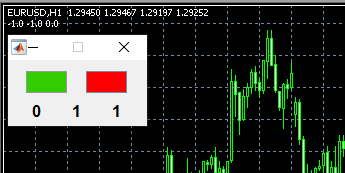14. NNM 变体

``` if(send1==false && Order1==1)
{
}

if(send1==true && Order1==0)
{
send1=false;
}

```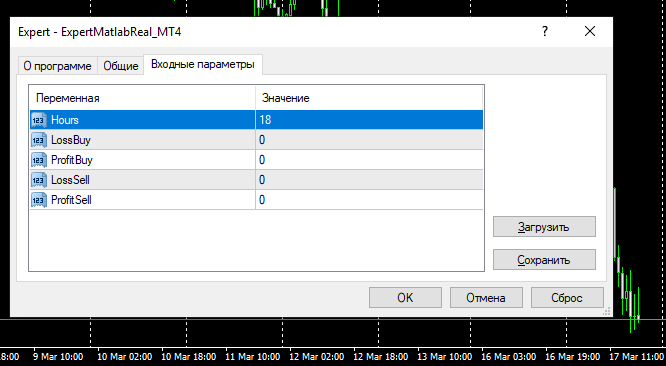14.1 外部变量 "Hours"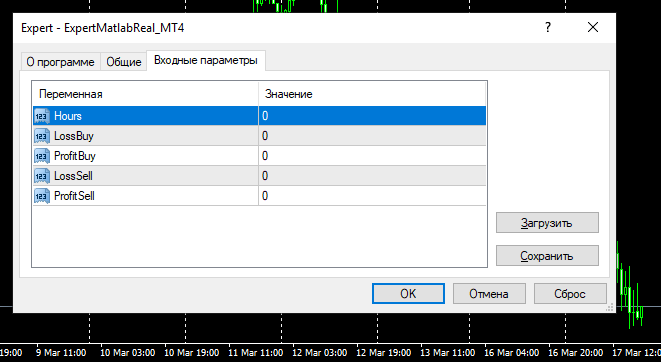14.2 Hours -0

2. 在可视模式里测试 NNM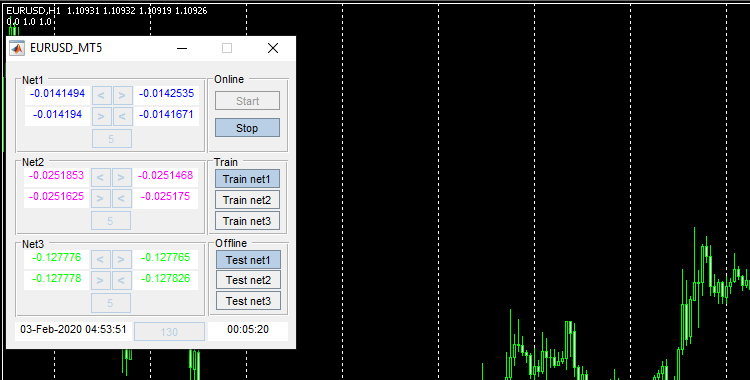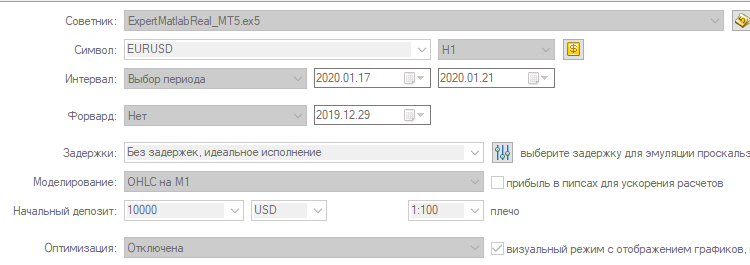15. 可视化模式

3. 神经网络训练

为了测试 Net1 子模块的两个神经网络，我们需要在 ...\Common\Files 目录中含有神经网络 net1-2，及含有训练数据的 EURUSDData.csv 文件。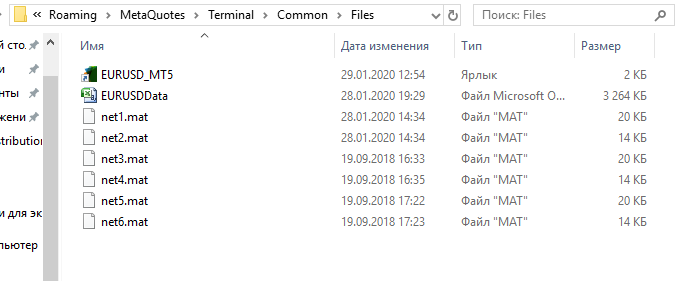16. 数据文件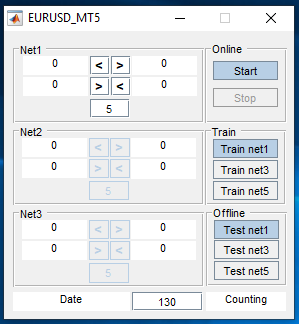17. 更新的按钮名称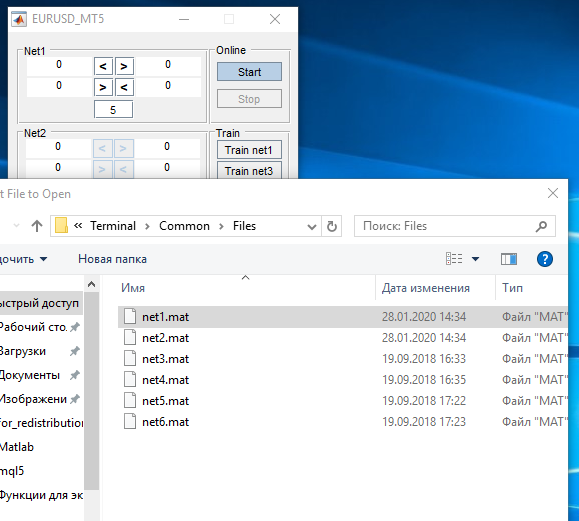18. Train net1

4. 从神经网络模块接收响应，以便优化交易策略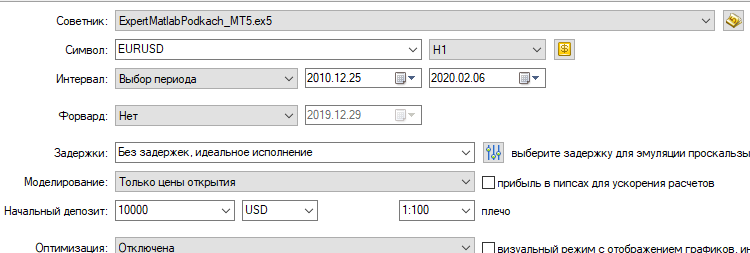19. 启动 ExpertMatlabPodkach_MT5.ex5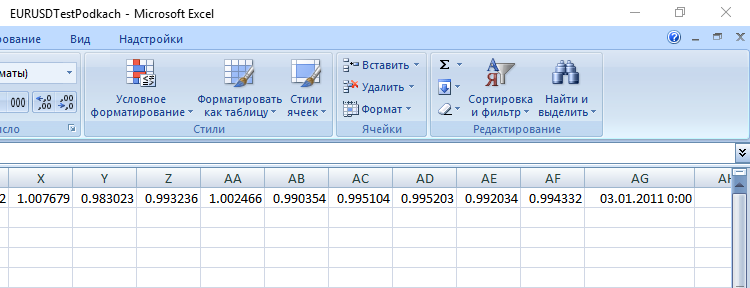20. 只留一行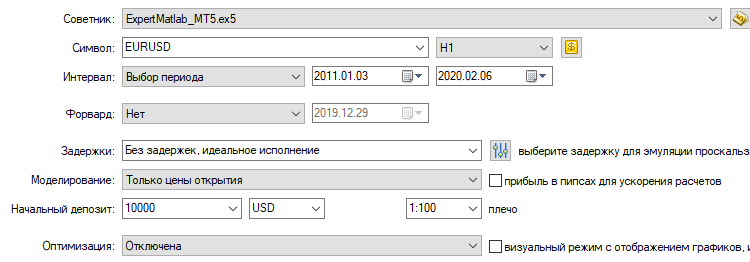21. 启动 ExpertMatlab_MT5.ex5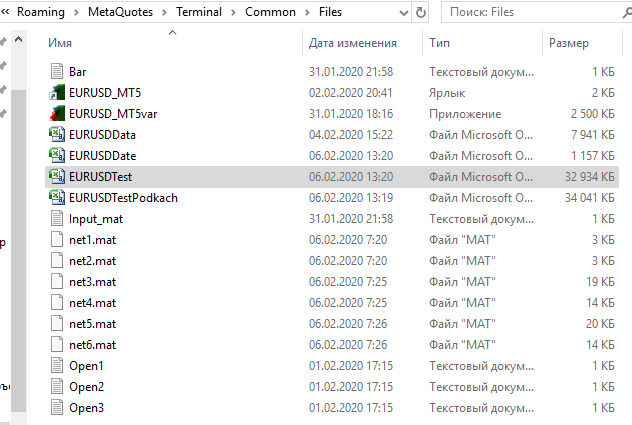22. 我们得到 EURUSDTest.csv 和 EURUSDDate.csv

ExpertMatlab_MT5.ex5 将生成两个测试文件 EURUSDDate.csv 和 EURUSDTest.csv。 启动神经网络模块，单击 “Test net1”，然后选择 net1.mat。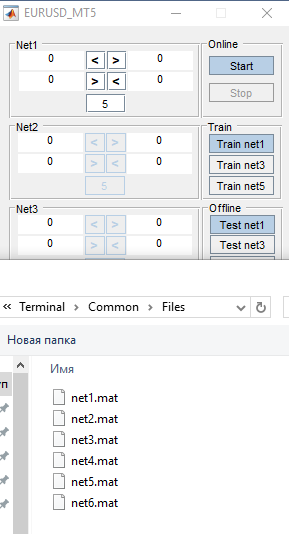23. 按下 "Test net1"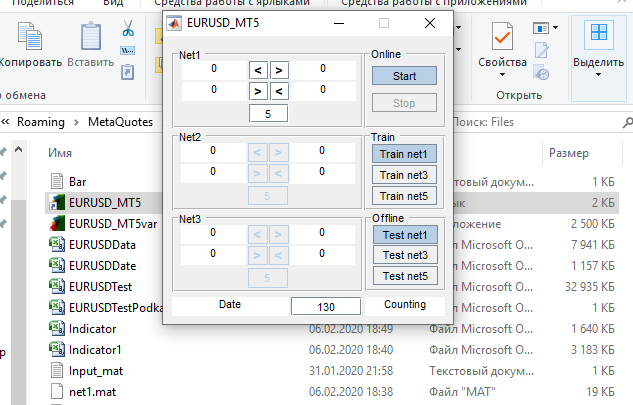24. 响应 Indicator1.csv 文件已生成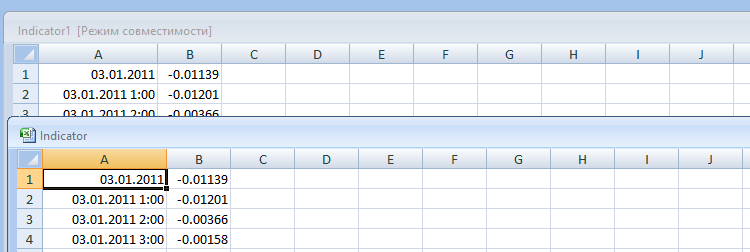25. 将 Indicator1.csv 另存为 Indicator.csv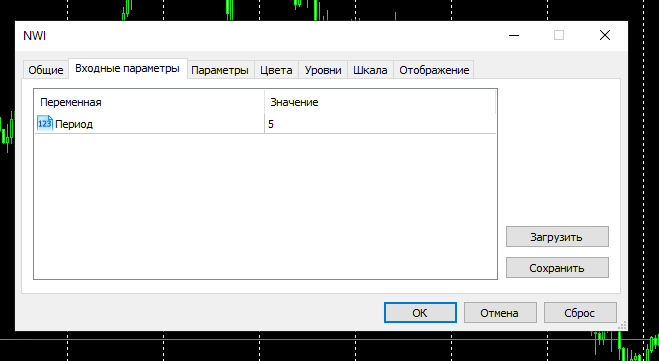26. 启动 NWI.ex5 指标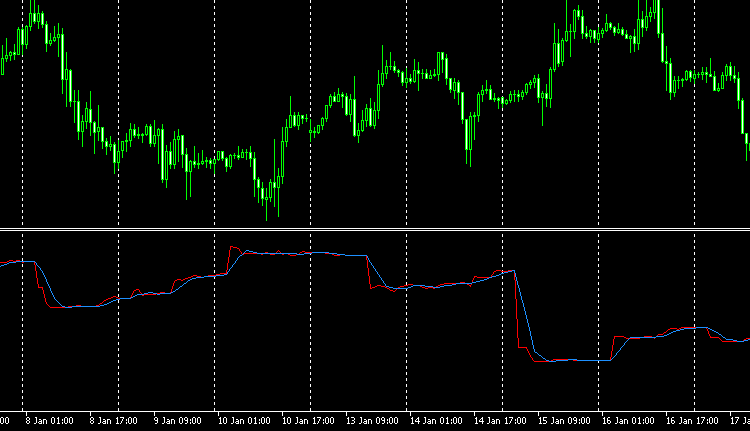27. NWI 可视化

```#include<Trade\Trade.mqh>

input int Period=5;
input int H1;
input int H2;
input int H3;
input int H4;

input int LossSell;
input int ProfitSell;

ulong TicketSell0;

datetime Count;

double Per;
double Buf_0[];
double Buf_1[];

bool send1;
bool send0;

int h=4;
int k;
int K;
int bars;
int Handle;
//+------------------------------------------------------------------+
//| Expert initialization function                                   |
//+------------------------------------------------------------------+
int OnInit()
{
//---

while(!FileIsEnding(Handle)&& !IsStopped())
{
bars++;
}
FileClose(Handle);

ArrayResize(Buf_0,bars);
ArrayResize(Buf_1,bars);

while(!FileIsEnding(Handle)&& !IsStopped())
{
h=Период-1;
if(k>=h)
{
while(h>=0)
{
Buf_1[k]=Buf_1[k]+Buf_0[k-h];
h--;
}
Buf_1[k]=Buf_1[k]/Period;
}
k++;
}
FileClose(Handle);

int deviation=10;

//---
return(INIT_SUCCEEDED);
}

//+------------------------------------------------------------------+
//| Expert deinitialization function                                 |
//+------------------------------------------------------------------+
void OnDeinit(const int reason)
{
//---

}
//+------------------------------------------------------------------+
//| Expert tick function                                             |
//+------------------------------------------------------------------+
void OnTick()
{
//---
MqlDateTime stm;
TimeToStruct(TimeCurrent(),stm);

int    digits=(int)SymbolInfoInteger(_Symbol,SYMBOL_DIGITS);
double point=SymbolInfoDouble(_Symbol,SYMBOL_POINT);
double PriceBid=SymbolInfoDouble(_Symbol,SYMBOL_BID);

double TP0=NormalizeDouble(PriceBid-ProfitSell*point,digits);

SL1=0;

TP1=0;

if(LossSell==0)
SL0=0;

if(ProfitSell==0)
TP0=0;

if(send1==false && K>0 && Buf_0[K-1]<Buf_1[K-1] && Buf_0[K]>Buf_1[K] && iLow(NULL,PERIOD_H1,1)<iLow(NULL,PERIOD_H1,2) && stm.hour>H1 && stm.hour<H2 && H1<H2)
{
}

if(send1==true && K>0 && Buf_0[K-1]>Buf_1[K-1] && Buf_0[K]<Buf_1[K] && iHigh(NULL,PERIOD_H1,1)>iHigh(NULL,PERIOD_H1,2))
{
send1=false;
}

//---------Sell0

if(send0==false && K>0 && Buf_0[K-1]>Buf_1[K-1] && Buf_0[K]<Buf_1[K] && iHigh(NULL,PERIOD_H1,1)>iHigh(NULL,PERIOD_H1,2) && stm.hour>H3 && stm.hour<H4 && H3<H4)
{
}

if(send0==true && K>0 && Buf_0[K-1]<Buf_1[K-1] && Buf_0[K]>Buf_1[K] && iLow(NULL,PERIOD_H1,1)<iLow(NULL,PERIOD_H1,2))
{
send0=false;
}
K++;
}

//+------------------------------------------------------------------+
//| Tester function                                                  |
//+------------------------------------------------------------------+
double OnTester()
{
//---
double ret=0.0;
//---

//---
return(ret);
}
//+------------------------------------------------------------------+

```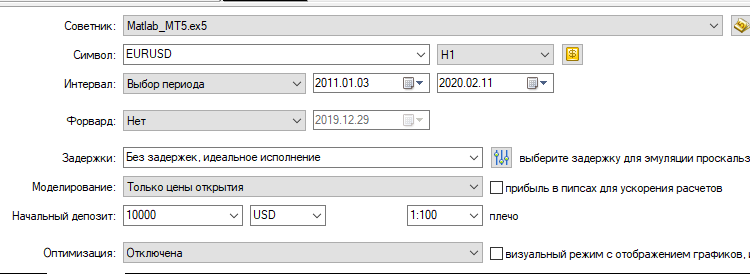28. 启动 Matlab_MT5.ex5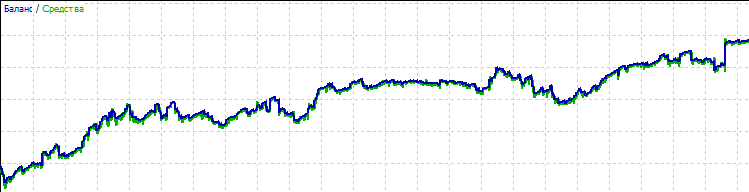29. 余额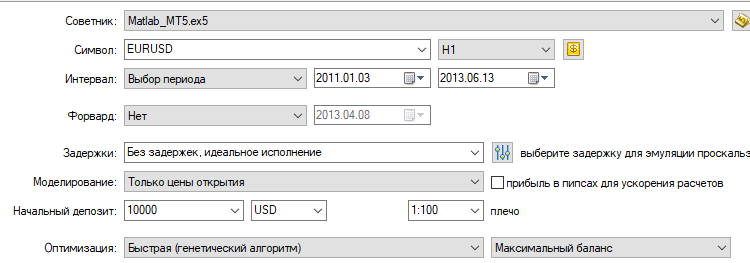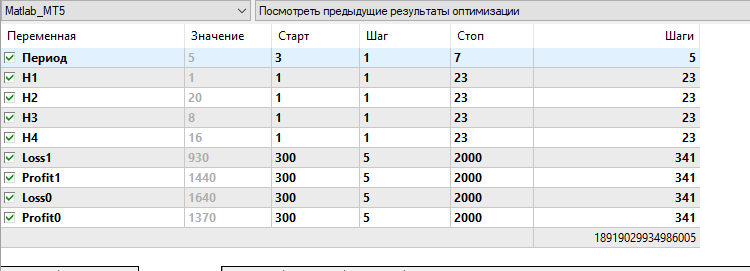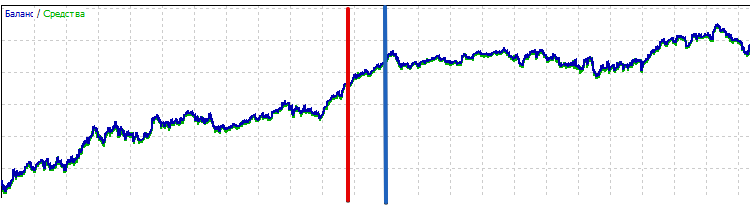30. 运行优化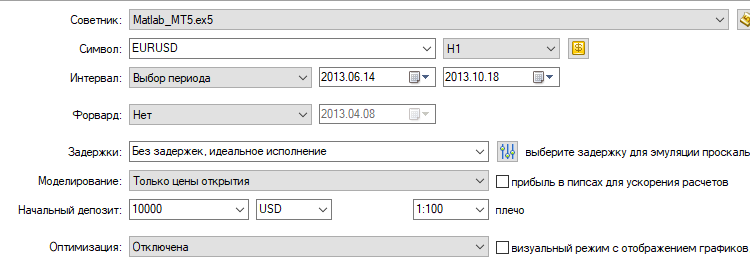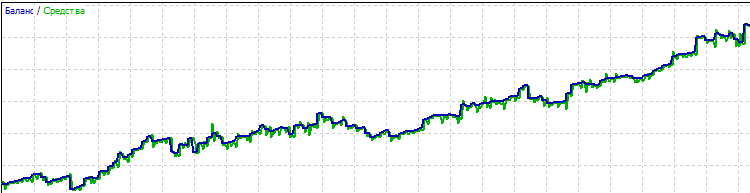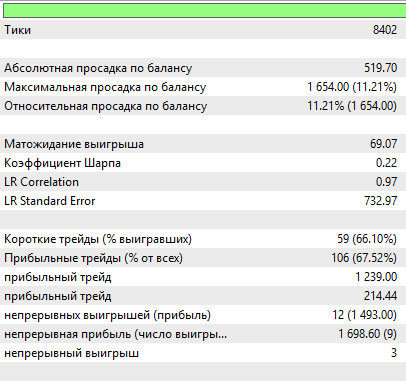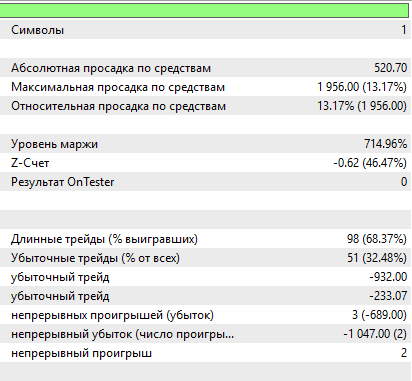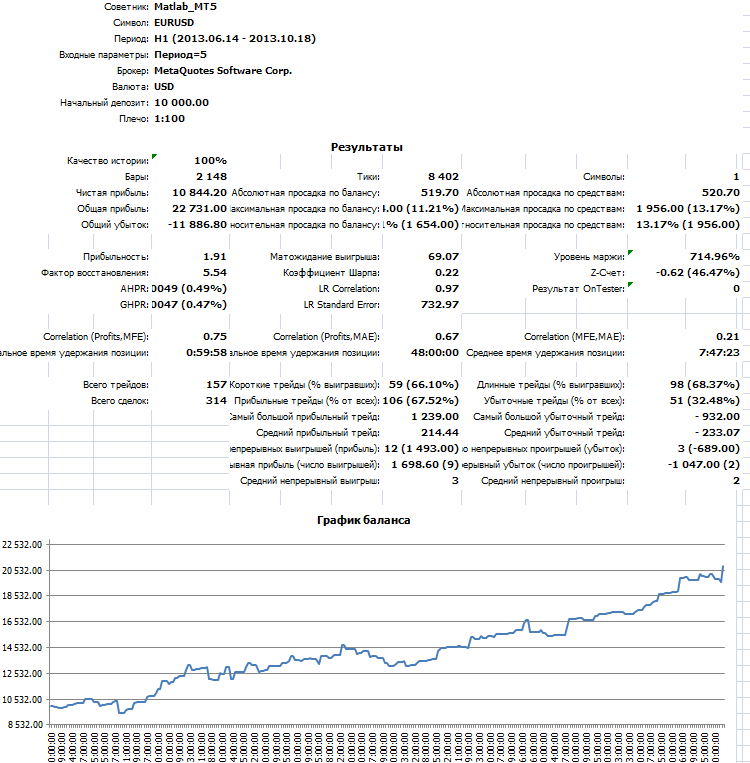31. 参考测试

5. 纠正错误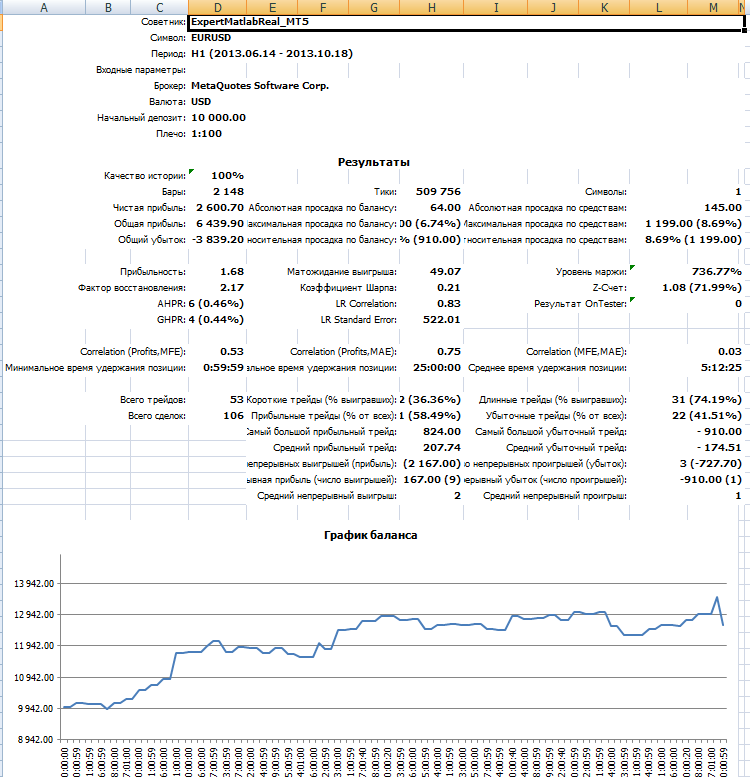32. 失败测试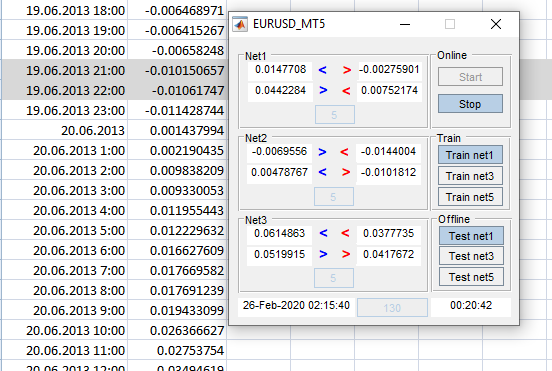33. 比较响应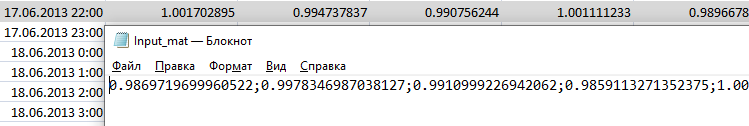34. 比较数据是否匹配

```ExpertMtatlab_MT5.ex5
if(stm.hour==0)
{
for(int i=19; i>=10; i--)
{
in[i-10]=in[i];
}

for(int i=29; i>=20; i--)
{
in[i-10]=in[i];
}
}
ExpertMatlabReal_MT5.ex5
if(Bar<Bars(NULL,PERIOD_H1) && stm.hour==0)
{
for(int i=19; i>=10; i--)
{
in[i-10]=in[i];
}

for(int i=29; i>=20; i--)
{
in[i-10]=in[i];
}
}

```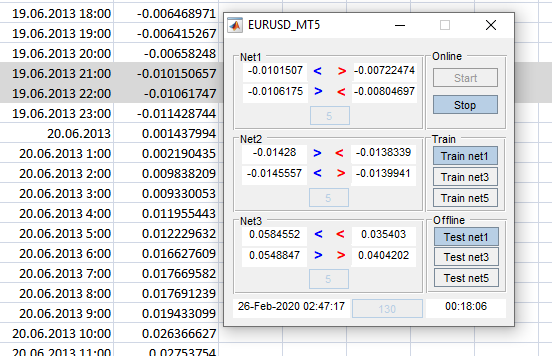35. 比较响应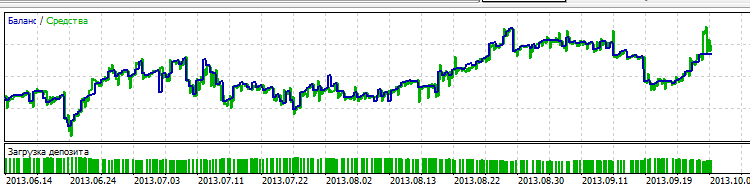36. 测试与参考不同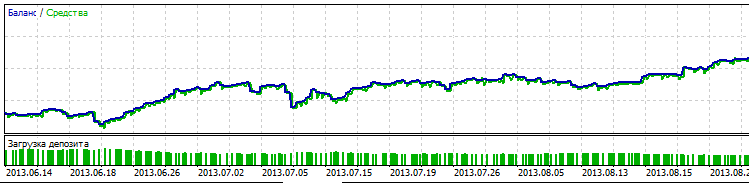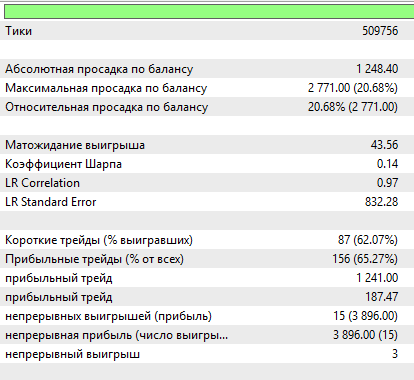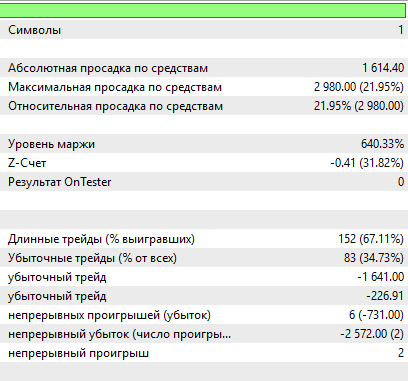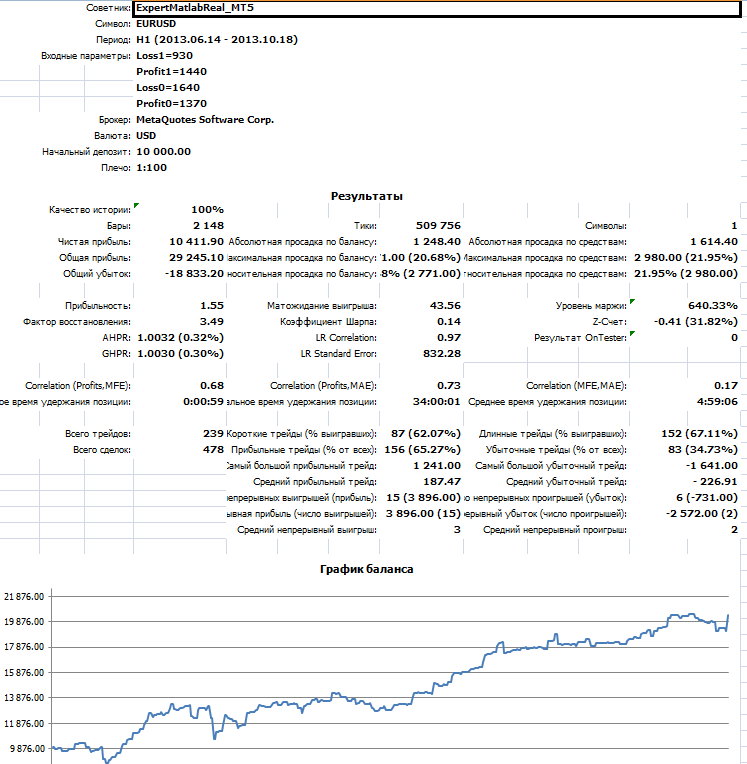37. 不等待即时报价进行测试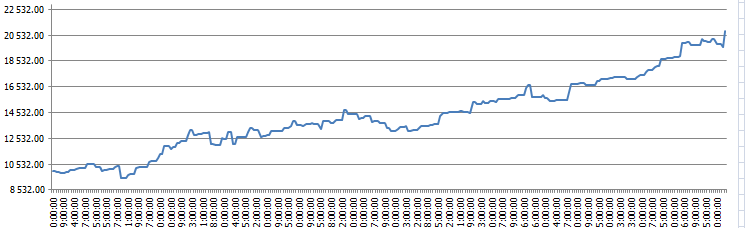38. 参考

``` if(Order1==0 && Bar<Bars(NULL,PERIOD_H1) && Con==0)
send0=true;

if(Order1==1 && Bar<Bars(NULL,PERIOD_H1) && Con==0)
send1=true;

```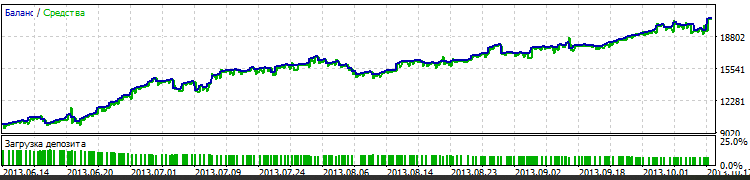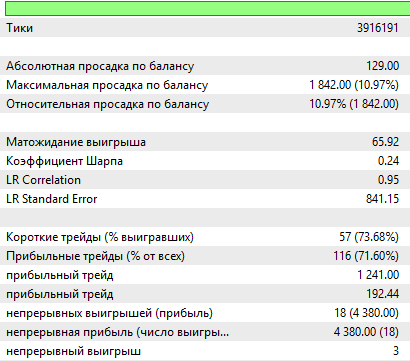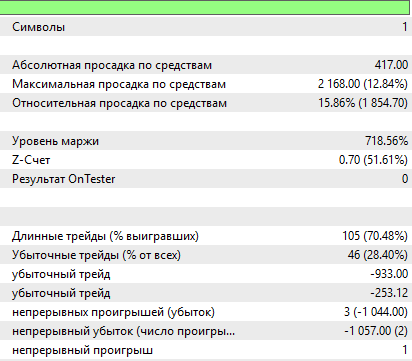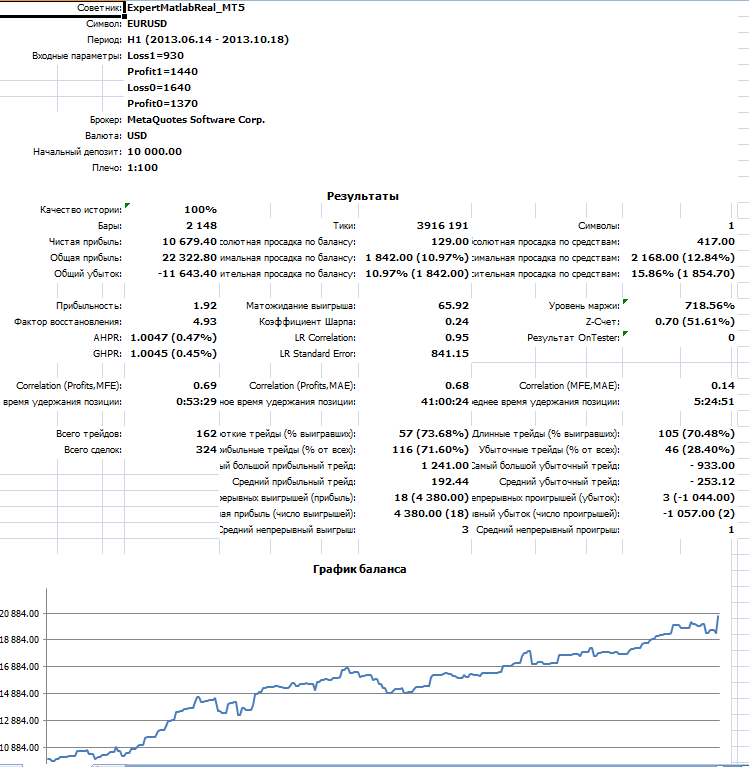39. 结果与参考接近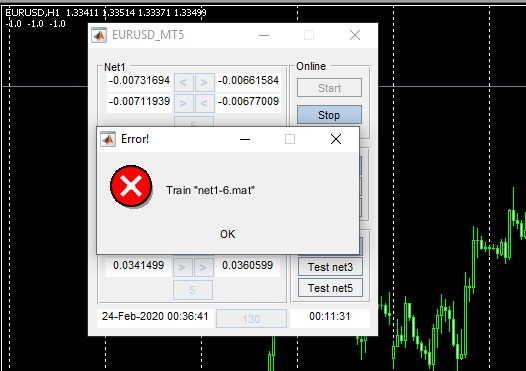40. 错误窗口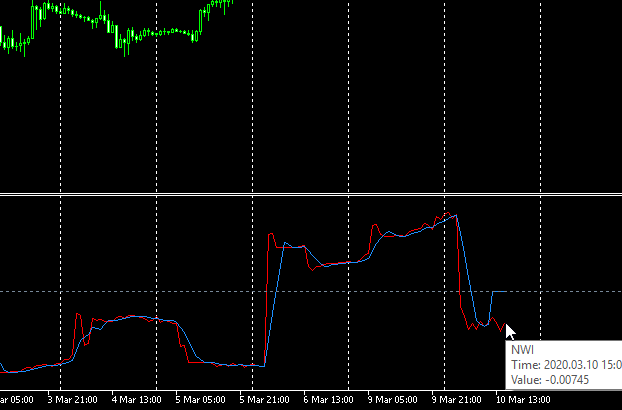41. MetaTrader 5 中的 NWI 指标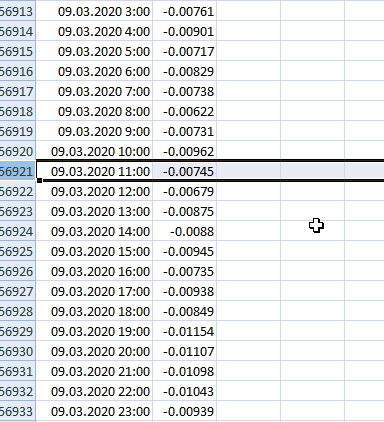42. 还有一个时移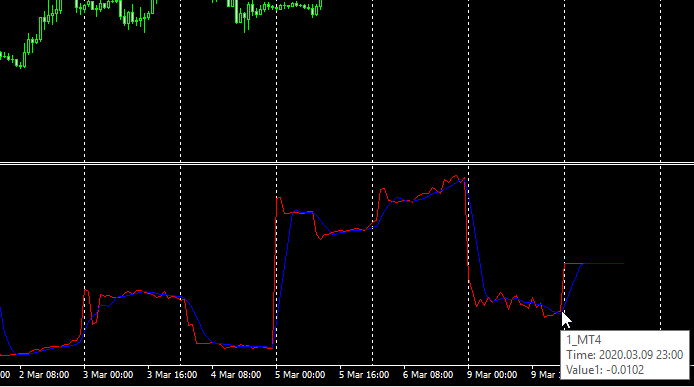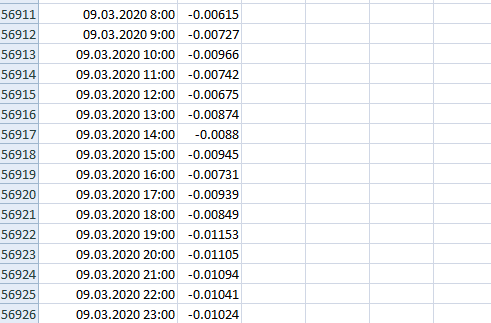44. 无偏移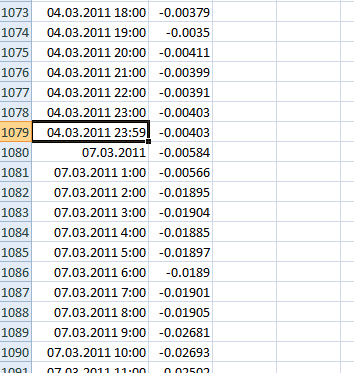45. 寻找偏移的原因

### 结束语

1. 神经网络能够在场外处理大量信息。
2. 由于信息是在离线模式下处理的，因此我们可以抢占市场先机，而不是跟在它后面跑。 或者至少我们可以跟上行情。
3. 结果就是，神经网络不仅可以在交易期间，甚至在准备阶段都能对行情状况的变化做出及时响应。 故此，在趋势变化，或因其他因素影响行情的情况下，我们可以冲抵大量亏损。
4. 至于本文中介绍的特定神经网络及其训练方法，毫无疑问，这种组合的优势在于，我们可以利用训练神经网络时得到的响应来优化 EA。 基于其他体系结构的神经网络能够在训练模式下表现出良好的结果，而在测试期间得结果却明显不同。 在我们的案例中，结果大致相同。 这令我们能够在当前日期附近测试 NN，并考虑历史上可用的市场因素。 本文未涵盖此部分，但您可以自行探索。

### Python

EURUSDData.csv (8009.95 KB)
Matlab_MT5.mq5 (9.36 KB)
NWI.mq5 (5.02 KB)

#### 该作者的其他文章| 28 12月 2020 在 12:29
Great job,I would like to try it.Btw,the english pannel will be easier to understand. After all,I don’t know your mother tongue.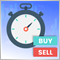快捷手动交易工具箱：基本功能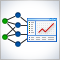神经网络在交易中的实际应用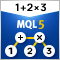计算数学表达式（第一部分）。 递归下降解析器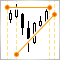手工图表和交易工具包（第一部分）。 准备：结构描述和助手类